### Home > A2C > Chapter 13 > Lesson 13.1.4 > Problem13-75

13-75.
1. Multiply the expressions in parts (a) through (d). Homework Help ✎

1. (a + b)(a2ab + b2)

2. (x − 2)(x2 + 2x + 4)

3. (y + 5)(y2 − 5y + 25)

4. (x − y)(x2 + xy + y2)

5. What did you notice about these products?

6. Make up another multiplication problem that will follow the same pattern.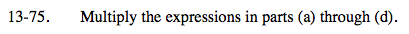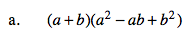a3 + b3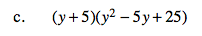See parts (a) and (b).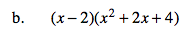x3 − 8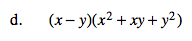See parts (a) and (b).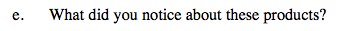What did they all have in common?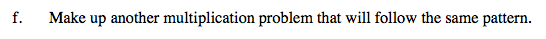Substitute values into the expression in either part (a) or part (d).